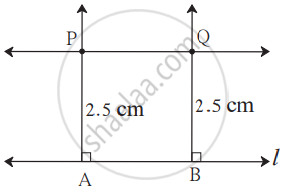# To draw a parallel line to a given line at a given distance:

Draw a line parallel to line l at a distance of 2.5 cm.### Steps of construction:

1. Draw line l.
2. Take two points A and B on the line l.
3. Draw perpendiculars to the line l from points A and B.
4. On the perpendicular lines take points P and Q at a distance of 2.5 cm from A and B respectively.
5. Draw line PQ.
6. Line PQ is a line parallel to the line l at a distance of 2.5 cm.
If you would like to contribute notes or other learning material, please submit them using the button below.

### Shaalaa.com

To Draw a Parallel Line to a Given Line at a Given Distance. [00:01:52]
S
0%